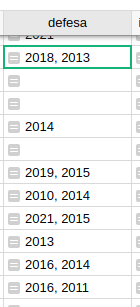# Calculating gap between years

Hi folks!

I have this column “defesa” that aggregate the year of post-graduation degree from Master and PhD.Which is created with:

``````values = [c.Defesa for c in Formacao.lookupRecords(Orientando=\$id) if c.Defesa]
return list(set(values))
``````

I would like to have another column that calculates the gap between the years. Something like:

``````if len(defesa)=2
gap = "year1" - "year2"
return gap
``````

Is it possible?

Hi Eduardo!

Here is the formula you are looking for:

``````if len(\$defesa)==2:
gap = abs(\$defesa-\$defesa)
return gap
``````

If there are two years listed under ‘Defesa’, find the difference between the two years then take the absolute value of that number (so it is always positive) - then return that number.

Let me know if you have any questions!

Thanks,
Natalie

1 Like

You are a STAR Natalie!Thanks! Perfect!

2 Likes